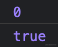# 信仰崩了？Preact 开始采用 Vue3 的响应式设计# 前言

`Performance`版的`React`

# Preact 简介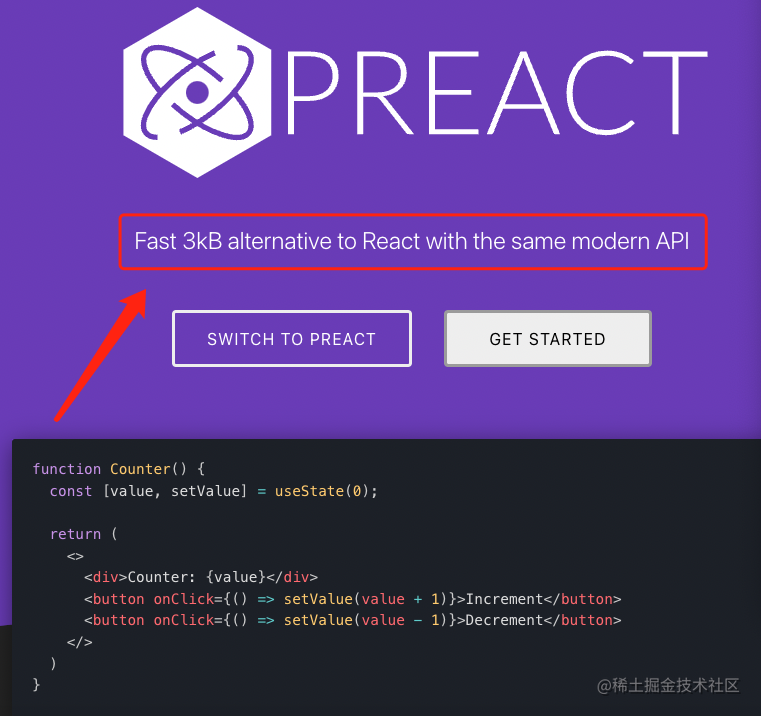``````npm create vite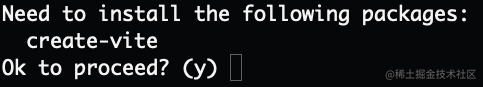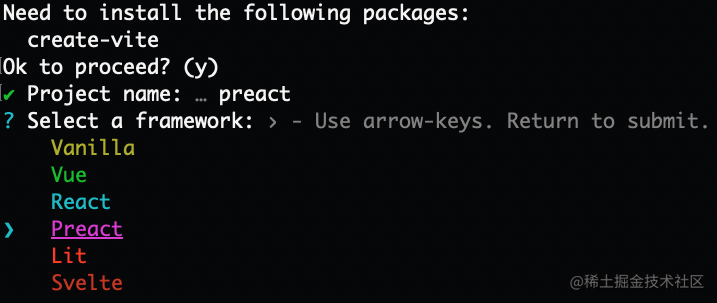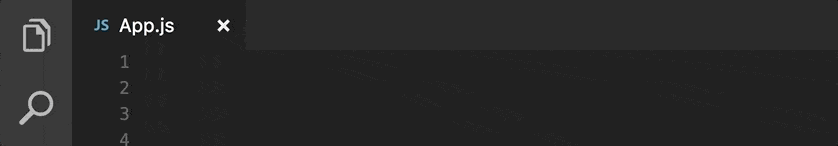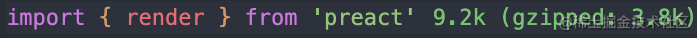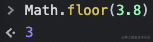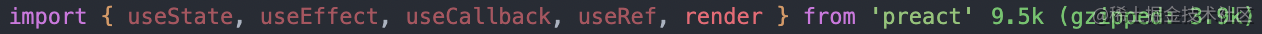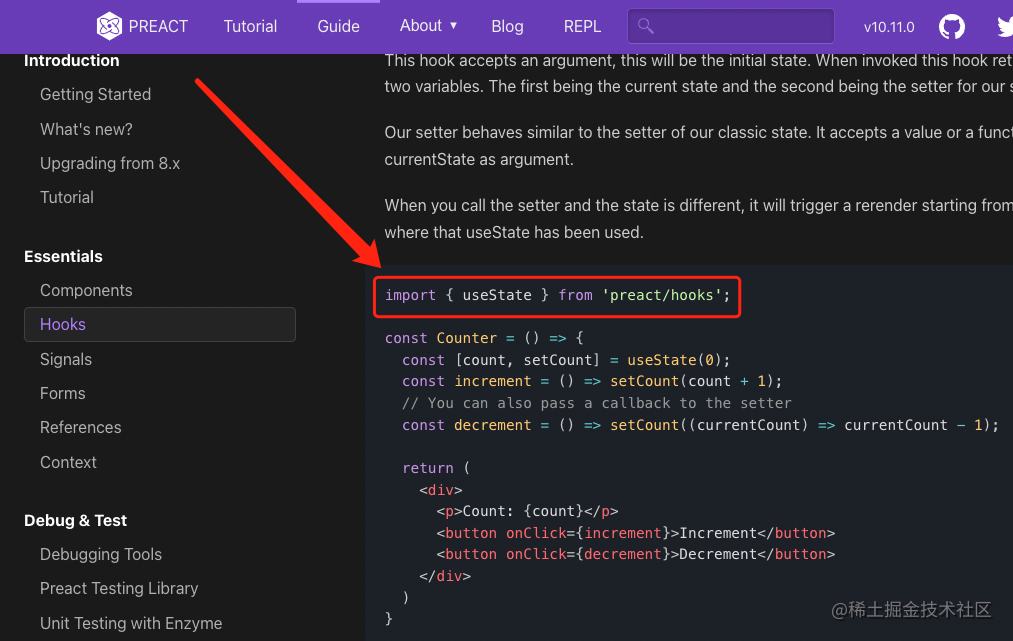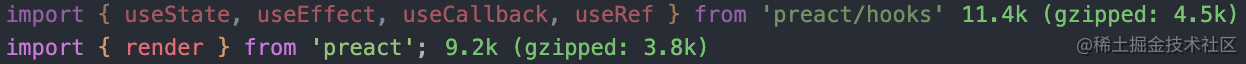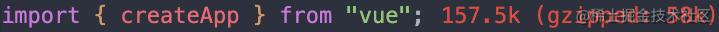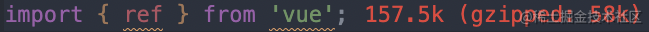# Preact Signals

``````import { signal, computed } from "@preact/signals";

const count = signal(0);
const double = computed(() => count.value * 2);

function Counter() {
return (
<button onClick={() => count.value++}>
{count} x 2 = {double}
</button>
);
}

``````<script setup>
import { ref, computed } from "vue";

const count = ref(0);
const double = computed(() => count.value * 2);
</script>

<template>
<button @click="count++">
{{count}} x 2 = {{double}}
</button>
</template>

`hooks`不同，`signals`可以在组件内部或外部使用。`signals`在类组件也可以很好的运行，因此您可以按照自己的节奏引入它们，并根据现状，在几个组件中试用它们，并随着时间的推移逐渐采用它们。—— `Preact团队`• 易冲突的全局状态
• 混乱的上下文
• 寻求更好的状态管理
• 卓越的性能• 避免了`React Hooks`的一些心智负担
• 性能比`React`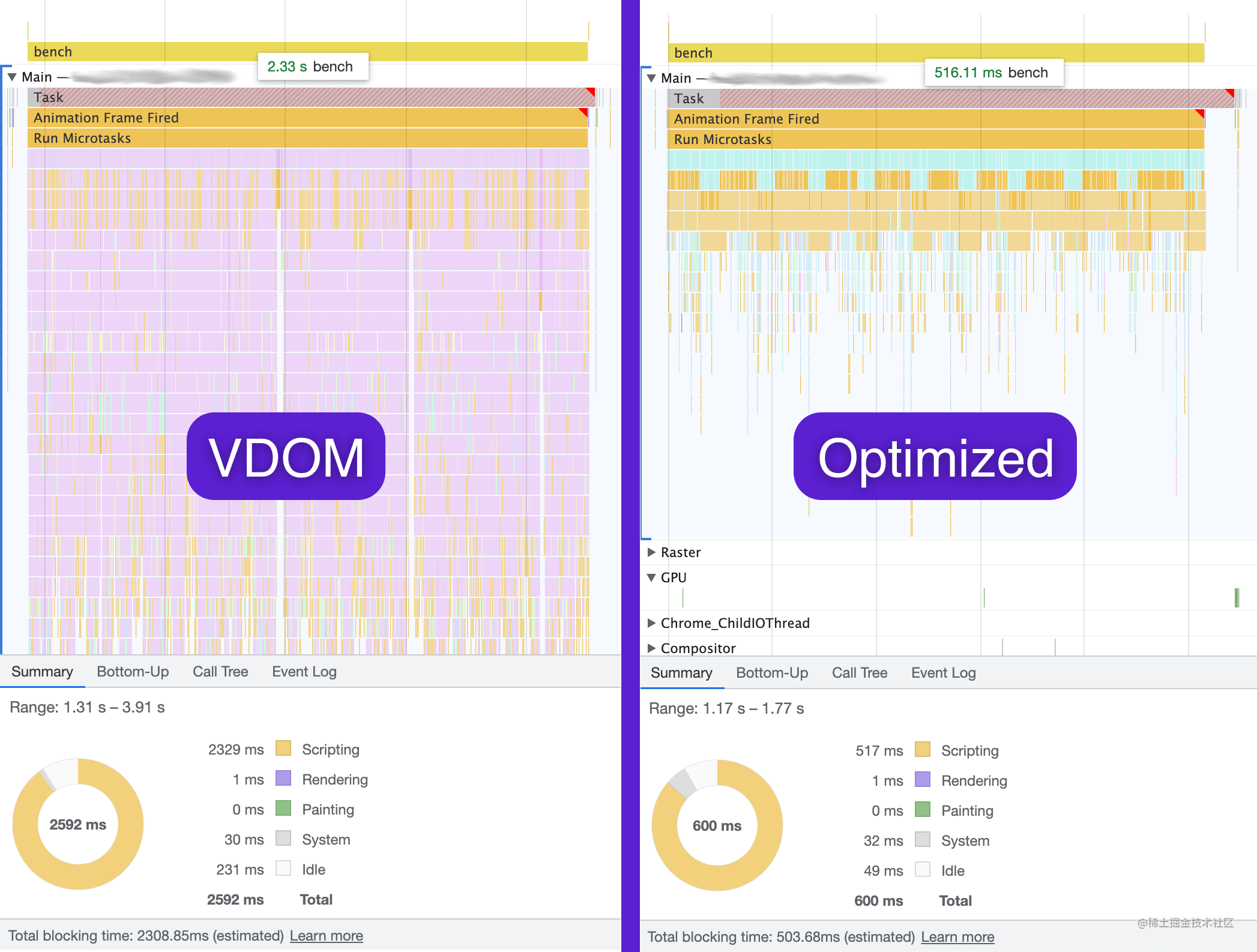`Preact`的老师`React`有在`React`里实现`Vue`的计划吗？答案是否定的，自从`Preact Signals`发布后大家就疯狂`@Dan``Dan`看完后直接来了句：这与`React`的发展理念不是很吻合。(潜台词：我们才不会在`React`里实现`Vue`呢)

`Angular`用装饰器用的好好的那是因为人家强制要求使用`TS`，但`Vue`显然不可能这样做。而且为了防止未来装饰器有变动（其实最近已经`Stage3`的装饰器已经和`TS`装饰器不一样了），许多曾经使用装饰器语法的库为了规避这个风险也已经改用了别的写法，如：`MobX``React DnD`等…# 用法

`Preact`版的`composition api`主要分为三个部分：

• `@preact/signals-core`
• `@preact/signals`
• `@preact/signals-react`

``````import { signal } from "@preact/signals-core";

const counter = signal(0);

// Read value from signal, logs: 0
console.log(counter.value);

// Write to a signal
counter.value = 1;

``````const counter = signal(0);
const effectCount = signal(0);

effect(() => {
console.log(counter.value);

// Whenever this effect is triggered, increase `effectCount`.
// But we don't want this signal to react to `effectCount`
effectCount.value = effectCount.peek() + 1;
});

`composition api``@vue/composition-api`又有啥区别呢？区别就是`composition api`只是一个概念，而`@vue/composition-api`是一个实现了`composition api`的项目。当初尤雨溪提出`composition api`的时候(那时候还不叫`composition api`，好像叫什么`functional base api`)遭到了大量质疑的声音，于是有个大佬就用`Vue2`现有的`API`实现了一版尤雨溪的提案，尤雨溪觉得这玩意非常不错！你们老喷我是因为你们没有体验过函数式的好，你们先用用试试，试完了保证你们直呼真香！于是联系该作者把`Vue2`版的`composition api`合并到`Vue`的仓库中并发布为`@vue/composition-api`。但谁也不会用爱发电对不，刚开始当个娱乐项目给你宣传了，时间一长也没啥收益，该作者也就不维护了。此时另一位大佬出现了，他说既然没人维护了那就交给我吧！他就是肝帝`AntFu`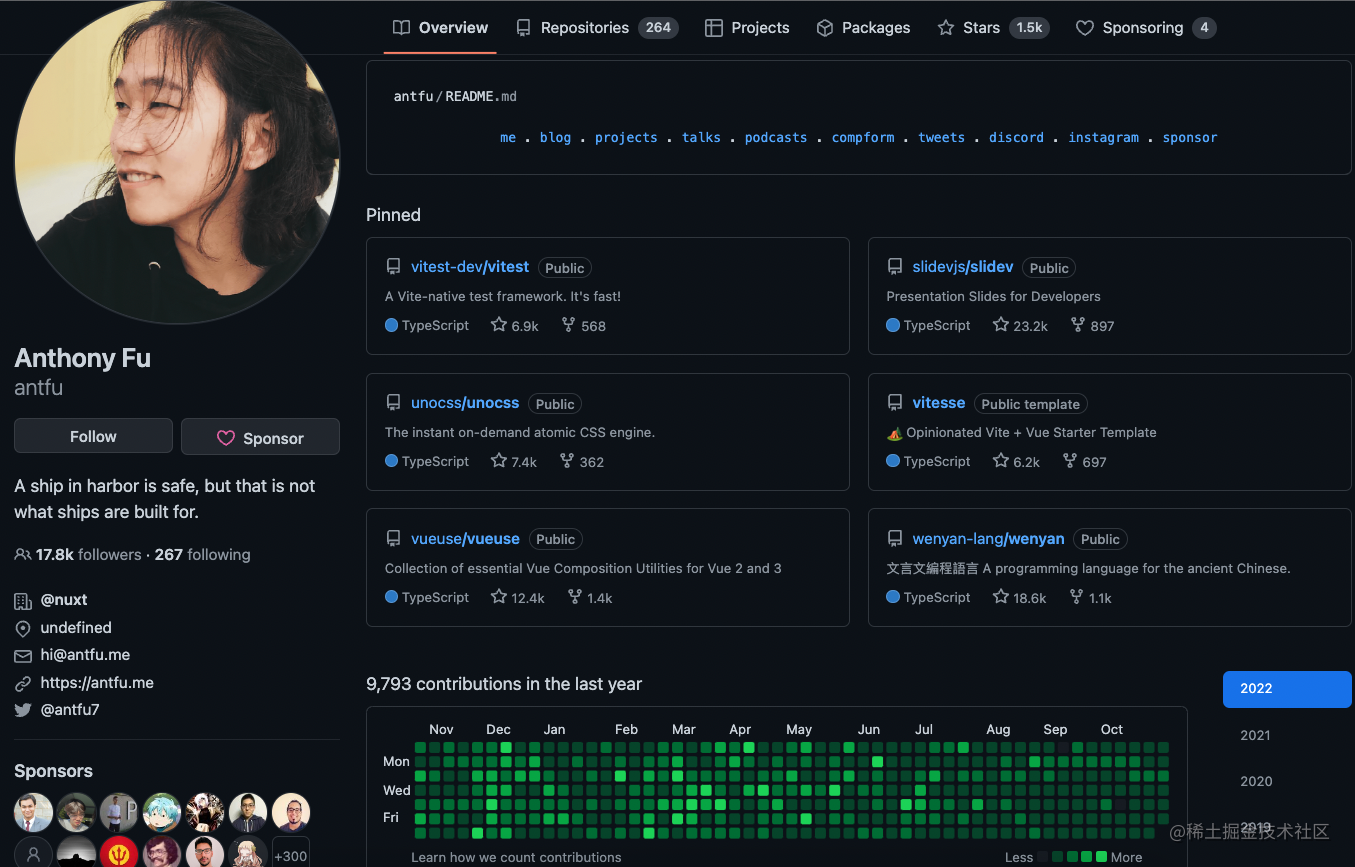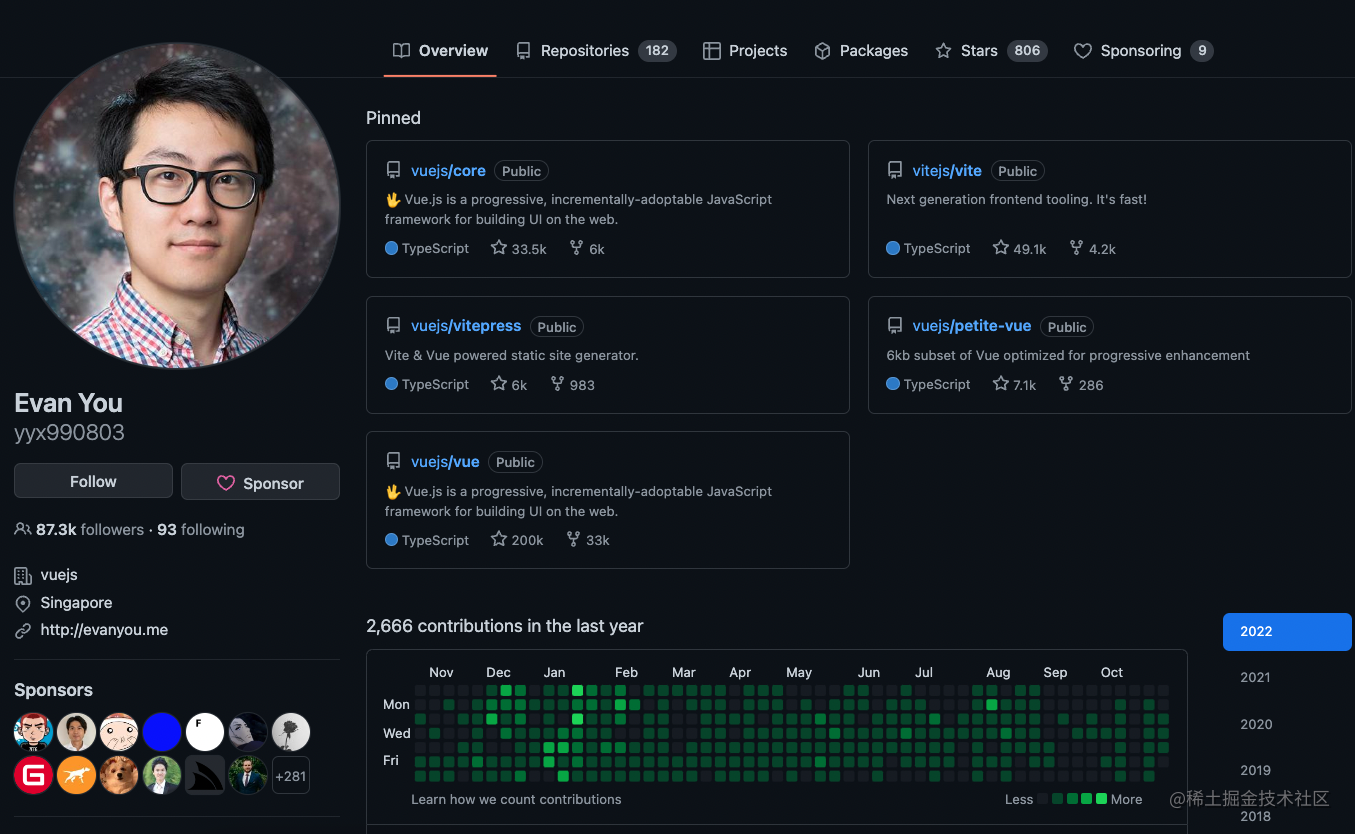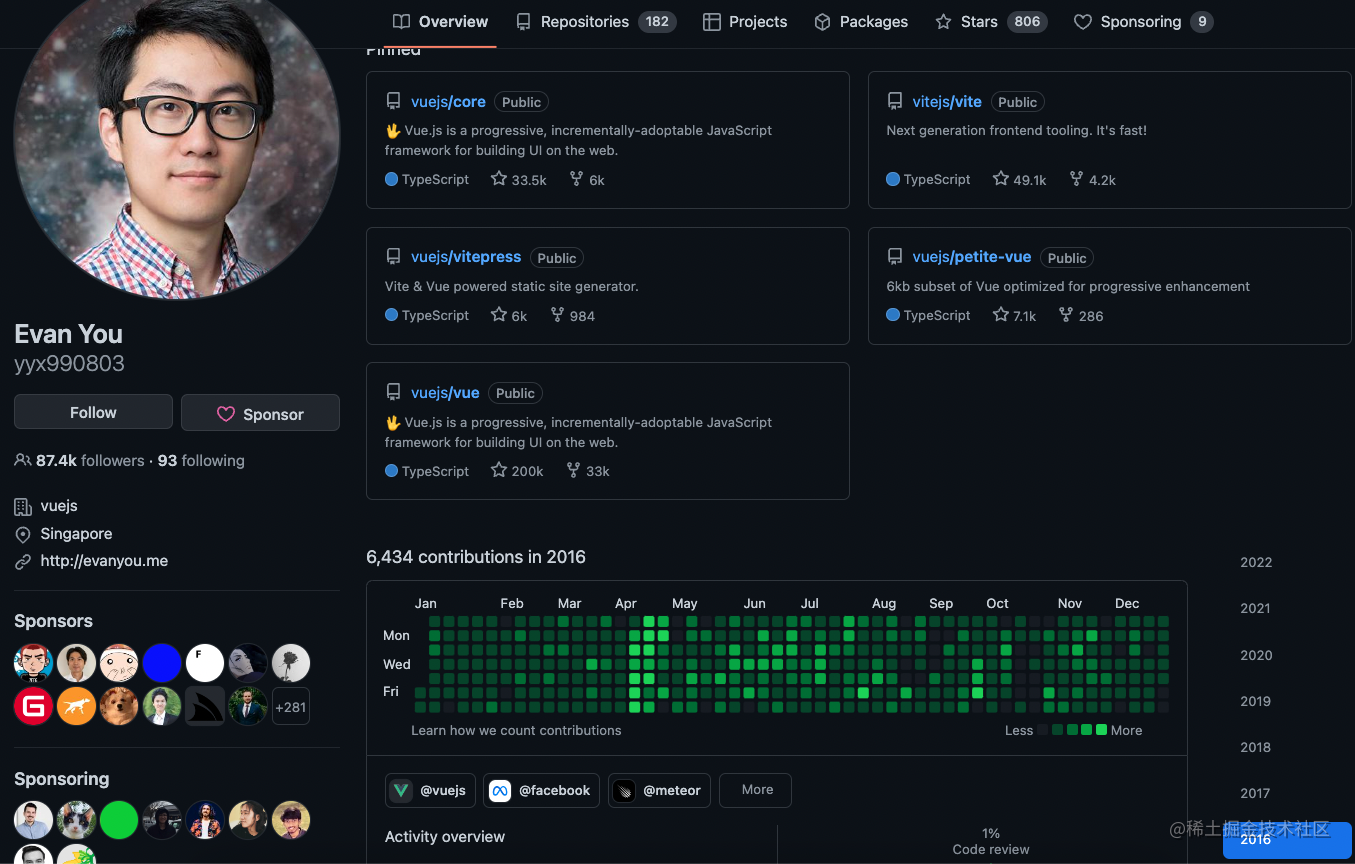``````import { ref, watchEffect } from 'vue';

const a = ref(0);
const b = ref(0);

watchEffect(() => {
console.log(a.value);

b.value++;
});

``````import { ref, watch } from 'vue';

const a = ref(0);
const b = ref(0);

watch(a, value => {
console.log(value);

b.value++;
});

``````import { ref, watch } from 'vue';

const a = ref(0);
const b = ref(0);

watch([a, b], ([valueA, valueB]) => {
console.log(valueA);

b.value = valueB + 1;
});

`Vue`的方案是既提供一个自动收集依赖的`watchEffect`，同时也提供一个手动收集依赖的`watch`

`Preact`的方案则是只提供一个`effect`(类似`Vue``watchEffect`)，如果你写出类似上面那样的代码：

``````import { signal, effect } from '@preact/signals-core';

const a = signal(0);
const b = signal(0);

effect(() => {
console.log(a.value);

b.value++;
});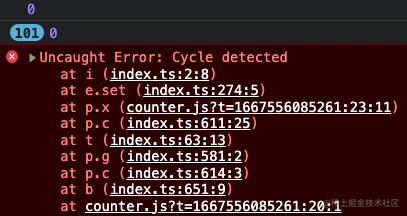《尤雨溪国外教程：亲手带你写个简易版的Vue！》

`Preact`没做这样的处理怎么办呢？那我们就避免在`effect`里既对`signal`进行取值操作同时又对它进行赋值操作呗！``````import { createSignal, createEffect, untrack } from "solid-js";

const [a, setA] = createSignal(0);
const [b, setB] = createSignal(0);

createEffect(() => {
console.log(a());
console.log(untrack(b));
});

``````import { signal, effect } from '@preact/signals-core';

const a = signal(0);
const b = signal(0);

effect(() => {
console.log(a.value);

b.value = b.peek() + 1;
});``````import { signal, computed } from "@preact/signals-core";

const name = signal("Jane");
const surname = signal("Doe");

const fullName = computed(() => name.value + " " + surname.value);

// Logs: "Jane Doe"
console.log(fullName.value);

// Updates flow through computed, but only if someone
// subscribes to it. More on that later.
name.value = "John";
// Logs: "John Doe"
console.log(fullName.value);

``````import { signal, computed, effect } from "@preact/signals-core";

const name = signal("Jane");
const surname = signal("Doe");
const fullName = computed(() => name.value + " " + surname.value);

// Logs: "Jane Doe"
effect(() => console.log(fullName.value));

// Updating one of its dependencies will automatically trigger
// the effect above, and will print "John Doe" to the console.
name.value = "John";

``````import { signal, computed, effect } from "@preact/signals-core";

const name = signal("Jane");
const surname = signal("Doe");
const fullName = computed(() => name.value + " " + surname.value);

// Logs: "Jane Doe"
const dispose = effect(() => console.log(fullName.value));

// Destroy effect and subscriptions
dispose();

// Update does nothing, because no one is subscribed anymore.
// Even the computed `fullName` signal won't change, because it knows
// that no one listens to it.
surname.value = "Doe 2";

``````import { signal, computed, effect, batch } from "@preact/signals-core";

const name = signal("Jane");
const surname = signal("Doe");
const fullName = computed(() => name.value + " " + surname.value);

// Logs: "Jane Doe"
effect(() => console.log(fullName.value));

// Combines both signal writes into one update. Once the callback
// returns the `effect` will trigger and we'll log "Foo Bar"
batch(() => {
name.value = "Foo";
surname.value = "Bar";
});

``````import { signal, effect } from "@preact/signals-core";

const a = signal(0);

effect(() => console.log(a.value));

a.value++;
a.value++;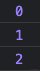``````import { ref,  watchEffect } from 'vue';

const a = ref(0);

watchEffect(() => console.log(a.value));

a.value++;
a.value++;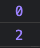``````import { ref,  effect } from '@vue/reactivity';

const a = ref(0);

effect(() => console.log(a.value));

a.value++;
a.value++;``````import { signal, effect, batch } from "@preact/signals-core";

const a = signal(0);

effect(() => console.log(a.value));

batch(() => {
a.value++;
a.value++;
})

`@vue/reactivity`中要想要达到同样效果的话… 关键是这个`@vue/reactivity`连个文档都没有！`Vue`官网上的`Composition API`是又封装了一层，用法已经不一样了。比方说`Composition API`里的`watch``@vue/reactivity`里就没有，而且`watchEffect``effect`表现也不太一致，`@vue/reactivity``README`写的也特别简陋：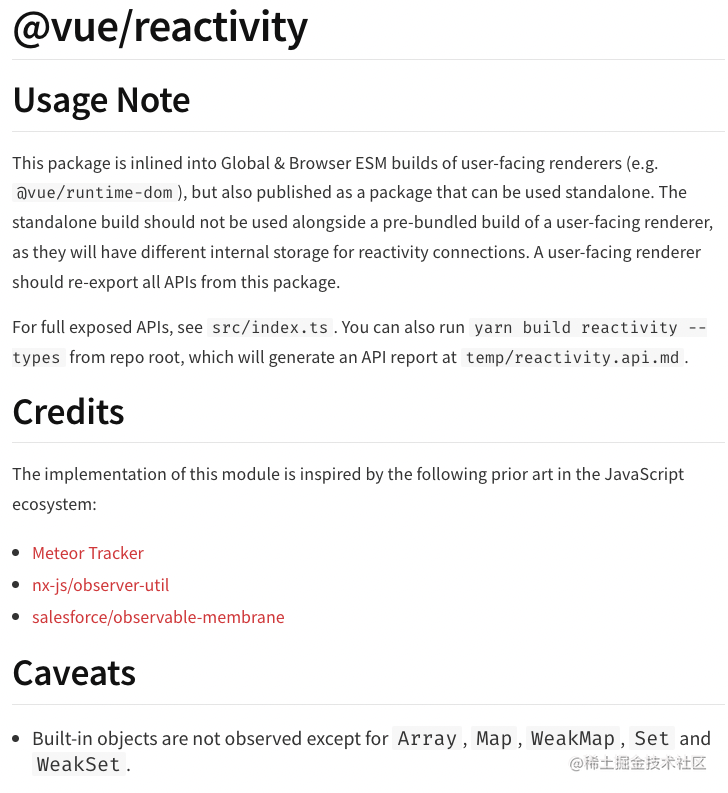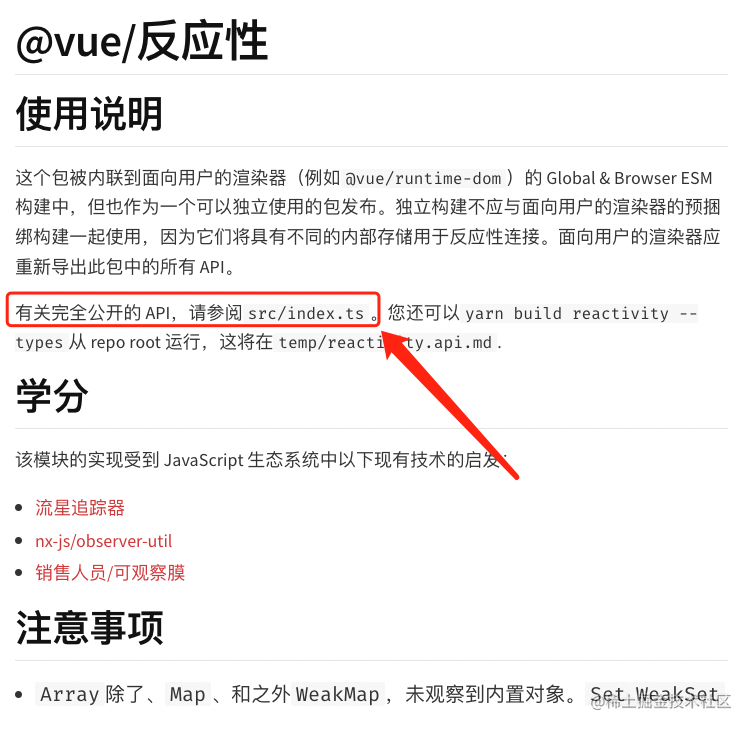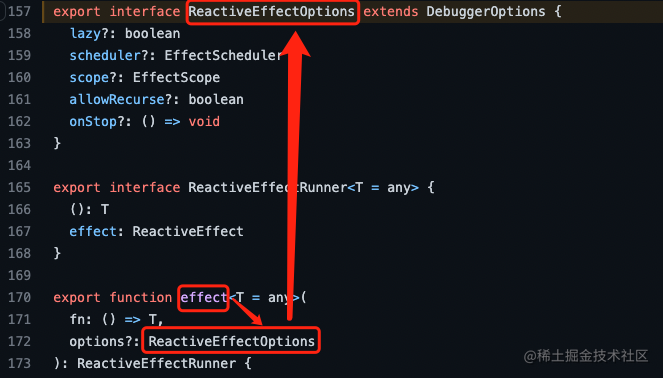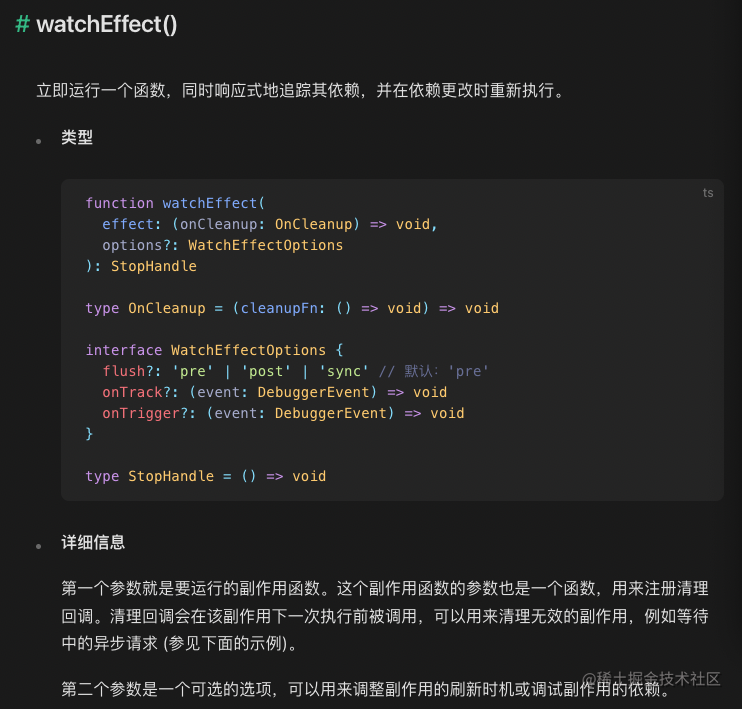``````import { ref, effect } from "@vue/reactivity";

const a = ref(0);

effect(() => console.log(a.value), { lazy: true });

a.value++;
a.value++;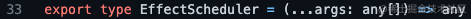``````import { signal, computed, effect, batch } from "@preact/signals-core";

const counter = signal(0);
const double = computed(() => counter.value * 2);
const tripple = computed(() => counter.value * 3);

effect(() => console.log(double.value, tripple.value));

batch(() => {
counter.value = 1;
// Logs: 2, despite being inside batch, but `tripple`
// will only update once the callback is complete
console.log(double.value);
});
// Now we reached the end of the batch and call the effect

`batch`函数还可以嵌套着写：

``````import { signal, computed, effect, batch } from "@preact/signals-core";

const counter = signal(0);
effect(() => console.log(counter.value));

batch(() => {
batch(() => {
// Signal is invalidated, but update is not flushed because
// we're still inside another batch
counter.value = 1;
});

// Still not updated...
});
// Now the callback completed and we'll trigger the effect.

## React 及 Preact

`core`的核心部分讲完了，那就继续看看`@preact/signals`以及`@preact/signals-react`吧！它俩用法都一样：

``````import { signal } from "@preact/signals-react";

const count = signal(0);

function CounterValue() {
// Whenver the `count` signal is updated, we'll
// re-render this component automatically for you
return <p>Value: {count.value}</p>;
}

``````import { useSignal, useComputed } from "@preact/signals-react";

function Counter() {
const count = useSignal(0);
const double = useComputed(() => count.value * 2);

return (
<button onClick={() => count.value++}>
Value: {count.value}, value x 2 = {double.value}
</button>
);
}

# 后续

``````import { ref, effect } from '@vue/reactivity'

const a = ref(0)
const fn = effect(() => console.log(a.value), { lazy: true })

a.value++
fn()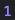``````import { ref, effect } from '@vue/reactivity'

const a = ref(0)
effect(
() => console.log(a.value),
{
scheduler (fn) {
console.log(fn)
}
}
)

a.value++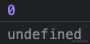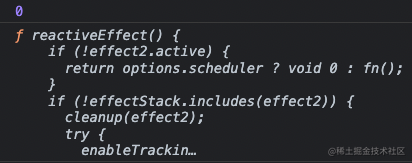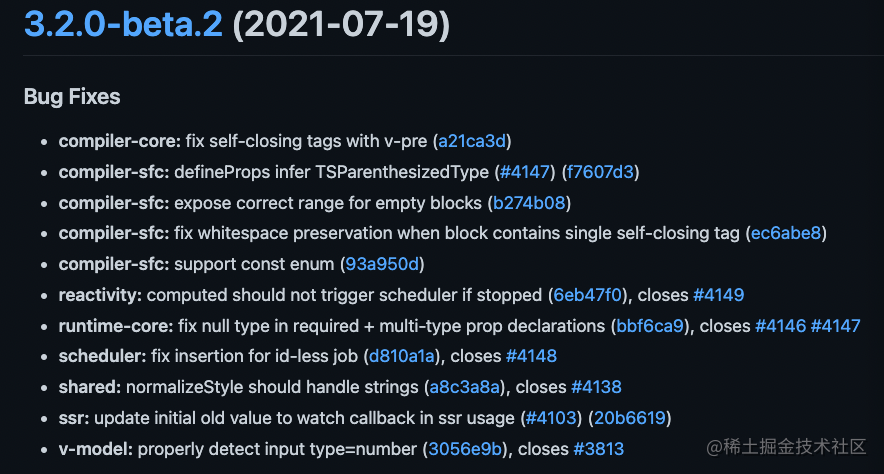``````// 3.2 以前
effect(
() => {},
{
scheduler (fn) {
console.log(fn)
}
}
)

// 3.2 之后（含 3.2）
const fn = effect(
() => {},
{
scheduler () {
console.log(fn)
}
}
)

``````import { ref, effect } from '@vue/reactivity'

const jobQueue = new Set()
const p = Promise.resolve()

let isFlushing = false
function flushJob() {
if (isFlushing) return

isFlushing = true

p.then(() => {
jobQueue.forEach(job => job())
}).finally(() => {
isFlushing = false
})
}

const a = ref(0)
const fn = effect(
() => console.log(a.value),
{
scheduler () {
flushJob()
}
}
)

a.value++
a.value++``````import { ref, effect } from '@vue/reactivity'

const a = ref(0)
const fn = effect(
() => console.log(a.value),
{
scheduler (func) {
console.log(fn === func)
}
}
)

a.value++